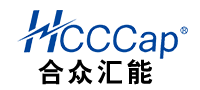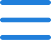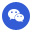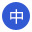400-088-5906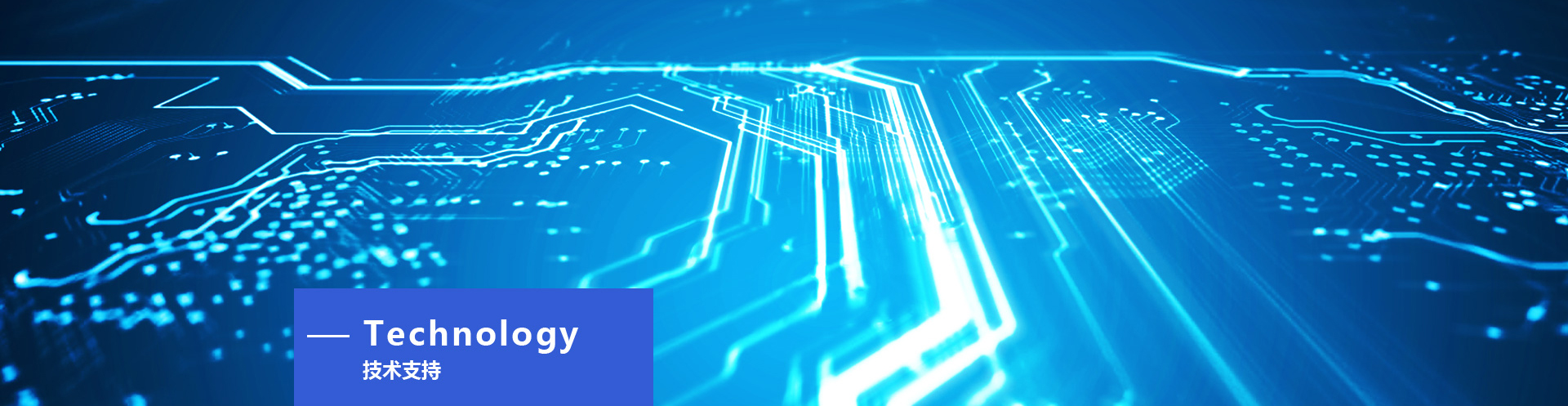Your location： Home > Technical Support
Performance test method
2015-05-07 735people visit

Capacity measurement

Supercapacitors show a significant "non-conductive absorption" energy storage mode, so the traditional method will not be able to accurately measure the capacity of supercapacitors, such as LCR meter commonly used in the laboratory, the measurement of the capacity of supercapacitors will be inaccurate. Below we list a measurement method of supercapacitor capacity, which is calculated based on RC time constant.

The following test circuit can be easily built in the laboratory, and the core is a power supply with constant current output and voltage limits as a charging power supply. The charging voltage waveform at both ends of the capacitor can be recorded by a digital oscilloscope. With the cursor of the oscilloscope, it is easy to read the time taken for the voltage to rise from 1.5v to 2.5v. The basic calculation formula is as follows: I =C (V/ t) the formula is converted to: C= I (t/ V). Charging current set to 1A, voltage range V= 2.5v -- 1.5v =1V then C= t, in this example, the capacitance of the supercapacitor is numerically equal to the time it takes the capacitor to charge from 1.5v to 2.5v. The unit of time is seconds.

Because of the particularity of ultracapacitor structure, the capacitor must be completely discharged before the test, so as to get more accurate results. If the ultracapacitor has been charged before the test, it is better to short-circuit the two terminals of the capacitor for more than 15 minutes to make the capacitor fully discharge.

Inflow current measurement

It is difficult to measure the actual self-discharge or self-leakage values of supercapacitors because of their obvious non-conductive properties. When an ultracapacitor is charged to the working voltage, the incoming current is large and gradually decreases. At this time, the inflow current is the sum of the dielectric absorption current and capacitor leakage current. The current absorbed by the medium is stored as energy, but deep storage takes a long time. The inflow current of the capacitor is logarithmic to the time, as shown in the following table.

These typical curves are measured by the circuit below, where the capacitance is short-circuited for two days before the measurement so that the stored energy is fully released.

Only when the dielectric absorption power supply is 0, the inflow current at this time is the leakage current, which needs about 72 hours of continuous charging to reach, at this time the leakage current is about a few microamps. After this point, in order to continue to measure the incoming current, an accurate microvoltmeter and a relatively large resistor are used, as shown in the figure above.

Dc impedance and ac impedance measurements

Internal Resistance and ESR (equlvalent series Resistance) Measurement

The series equivalent impedance or ac impedance can be measured at 1KHZ using the LCR bridge. The measurement result of this method is more accurate. The other method is to measure the so-called dc impedance. The same instrument can be used.

Below is made of capacitance measurement circuit for charging voltage curve, the starting stage of partial enlarged diagram as shown in the figure below, when used for charging current of 1 a, instantaneous voltage step can be used to calculate the mainstream resistance, DC resistance is equal to the step voltage or DC impedance values divided by the current of 1 a, for low resistance capacitance, can use a larger current measurements.

In this example, the internal resistance = initial voltage step/charging current = 0.15 V / 1 a = 0.15 Ω study, dc resistance is between 1.1 1.5 times that of the ac impedance.

Instructions：

1.The above information is for reference only. If you have any questions, you can consult the HCCCap Technology Department.

2.Data parameters are for reference only. The actual product parameters produced in different batches and at different times may change. Subject to the product actually received.The exact parameters should be checked with the manufacturer in time.

Telephone: 010-82897371
Email: hccenergy@gmail.com